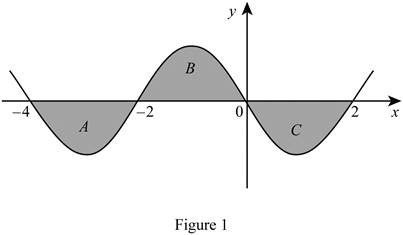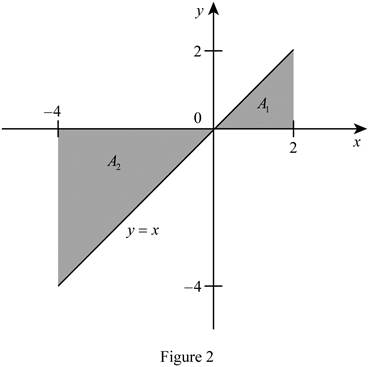# The value of the integral function ∫ − 4 2 [ f ( x ) + 2 x + 5 ] d x .### Single Variable Calculus: Concepts...

4th Edition
James Stewart
Publisher: Cengage Learning
ISBN: 9781337687805### Single Variable Calculus: Concepts...

4th Edition
James Stewart
Publisher: Cengage Learning
ISBN: 9781337687805

#### Solutions

Chapter 5.2, Problem 49E
To determine

## To calculate: The value of the integral function ∫−42[f(x)+2x+5]dx.

Expert Solution

The value of the integral function 42[f(x)+2x+5]dx is 15.

### Explanation of Solution

Given information:

The integral function is 42[f(x)+2x+5]dx (1)

The region lies between x=4 and x=2.

Calculation:

The integral property 2 is shown below:

ab[f(x)+g(x)]dx=abf(x)dx+abg(x)dx (2)

The property is valid only, if the functions f(x) and g(x) are continuous.

Apply the integral property 2 in Equation (1).

42[f(x)+2x+5]dx=42f(x)dx+242xdx+425dx (3)

Rearrange Equation (3) as shown below.

42[f(x)+2x+5]dx=I1+2I2+I3 (4)

Consider the regions A, B, and C bounded by the graph of f as in Figure 1.Refer to Figure 1.

The value of the region which lies in the integral 42f(x)dx is 3.

The value of the region which lies in the integral 20f(x)dx is 3.

The value of the region which lies in the integral 02f(x)dx is 3.

Calculate the integral I1 by rearranging the integral function 42f(x)dx as shown below:

I1=42f(x)dx+20f(x)dx+02f(x)dx=3+33=3

Calculate I2 using the relation:

I2=42xdx (5)

Consider the function g(x)=x.

The function g(x) represents a straight line with slope 1.

Plot the function g(x) within the limits [4,2] as shown in Figure 2.Refer to Figure 2.

Take the area bounded by the function g(x) within the limits [4,2] as A1 and A2

Modify Equation (5) using the Figure 2.

I2=42xdx=A1+A2=(12×2×2)(12×4×4)=6

Calculate I3 using the relation:

I2=425dx=5[x]42=5(2(4))=30

Calculate the integral function using Equation (4).

Substitute 3 for I1, 6 for I2 and 30 for I3 in Equation (4).

42[f(x)+2x+5]dx=I1+2I2+I3=3+2(6)+30=312+30=15

Therefore, the value of the integral function is 15.

### Have a homework question?

Subscribe to bartleby learn! Ask subject matter experts 30 homework questions each month. Plus, you’ll have access to millions of step-by-step textbook answers!